# Order relation

(diff) ← Older revision | Latest revision (diff) | Newer revision → (diff)

comparison of functions,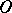-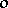relations, asymptotic relations

A notion arising in studies on the behaviour of a function with respect to another function in a neighbourhood of some point (this point may be infinite).

Letbe a limit point of a set. If for two functionsandthere exist constants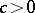and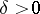such that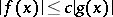for,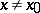, thenis called a function which is bounded in comparison within some deleted neighbourhood of, and this is written as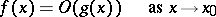(read "f is of the order of g" );means that the considered property holds only in some deleted neighbourhood of. This definition can be naturally used when,.

If two functionsandare such thatandas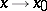, then they are called functions of the same order as. For instance, if two functionsare such that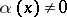,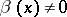ifand if the limit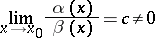exists, then they are of the same order as.

Two functionsandare called equivalent (asymptotically equal) as(written as) if in some neighbourhood of, except maybe the pointitself, a functionis defined such that(*)

The condition of equivalency of two functions is symmetric, i.e. if, thenas, and transitive, i.e. ifand, thenas. If in some neighbourhood of the pointthe inequalities,hold for, then (*) is equivalent to any of the following conditions: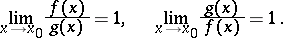Ifwhere, thenis said to be an infinitely-small function with respect to, and one writes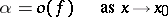(read "a is of lower order than f" ). If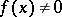when, thenif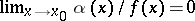. Ifis an infinitely-small function for, one says that the functionis an infinitely-small function of higher order thanas. Ifand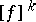are quantities of the same order, then one says thatis a quantity of orderwith respect to. All formulas of the above type are called asymptotic estimates; they are especially interesting for infinitely-small and infinitely-large functions.

Examples: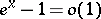(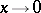);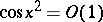;(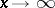;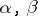any positive numbers);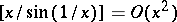().

Here are some properties of the symbolsand: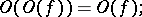if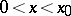and, then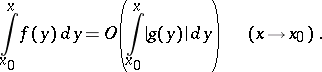Formulas containing the symbolsandare read only from the left to the right; however, this does not exclude that certain formulas remain true when read from the right to the left. The symbolsandfor functions of a complex variable and for functions of several variables are introduced in the same way as it was done above for functions of one real variable.

The symbolsand( "little oh symbollittle oh" and "big Oh symbolbig Oh" ) were introduced by E. Landau.